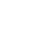×Want to shop in US Dollar? Try our Webshop specifically for North America!

# Rammstein Sneaker ”Logo Allover”

••40.00 EUR
In stock.

Black sneaker with grey Rammstein logos allover. Black laces and Rammstein lettering on the heel.

UK - EU - US

3 = 36 = 5
3.5 = 37 = 5.5

4 = 38 = 6
5 = 39 = 7
6 = 40 =7.5
7 = 41 = 8.5
8 = 42 = 9
9 = 43 =10
10 = 44 = 10.5
11 = 45 = 11.5
12 = 46 = 12
13 = 47 = 13

RAMMSTEINSHOP RECOMMENDS